## OpenDRO User's Guide

### 5.3 Status Menu

Note that the status menu is displayed on screen as "StAtUS".  The on-screen display for each menu item is shown underneath the menu name.

Item Machine Description
Tachometer
"tACh"
Both

Selecting this function will toggle the tachometer display off and on.  You are first asked to enter the desired units for the tachometer display either in RPM or in surface rate.  You then select the axis line to replace with the tachometer reading.

The surface rate is calculated with the RPM reading from the tachometer and the currently selected tool diameter and calculates the surface rate with the equation Surface Rate = (RPM * 2 * PI * radius).

Feed Rate
"FEEd"
Both

Display the instantaneous rate of movement per second for an axis.  After initiating this function, the DRO will prompt you to select an axis to display the rate of.  It will then ask for the line to display the feed rate on.  The axis position display for the selected line is replaced by the feed rate display for the previously selected axis.  This allows for simultaneously displaying both the position reading and rate for an axis.

When you move the axis, the rate is displayed for the axis relative to the current units.  For example, if the DRO is in metric mode and you move the axis at the rate of 5mm per second, then the display will show 5.0.  When you stop moving the axis, it will display 0.0.

To turn off the feed rate display, initiate this function again and the line displaying the feed rate will return to displaying the position reading for its axis.

Calculator
"CALC"
Both

Enter the reverse polish notation (RPN) calculator function.  The RPN calculator has a 4-level stack and supports basic mathematical functions and a number of special operations.

 Key Function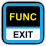Exit Exit the calculator and return to normal DRO function.  When the operation menu is displayed, this exits operation selection and returns to normal calculator function.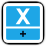Addition (+) Add the current and penultimate stack values together and push the result back on the stack.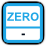Subtraction (-) Subtract the current stack value from the penultimate stack value and push the result back on the stack.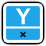Multiply (*) Multiply the current and penultimate stack values together and push the result back on the stack.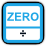Divide (/) Divide the penultimate stack value (dividend) from the current stack value (divisor) and push the result back on the stack.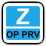Operation Previous Move to the previous operation in the list (see below).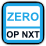Operation Next Move to the next operation in the list (see below).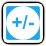Sign (+/-) Toggle the sign for the current stack value making a positive number negative and vice versa.Exit Leave calculator mode and return to DRO processing.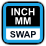Swap  Exchange the values for the current and penultimate positions in the stack.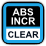Clear  Erase all stack values and set them to zero.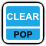Pop  Remove the current stack value and shift the stack down.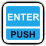Push  Push the current value onto the stack.  The next number entered will shift the stack up and start a new current value.  When the operation menu is active, this serves as a confirmation to execute the displayed operation

Example:

To divide 2.4 by 3, enter the following keys: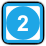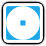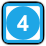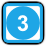The result of 0.8 is now the current value on the stack.

#### Operations

The calculator supports a number of mathematical operations in addition to the basics of addition, subtraction, multiplication, and division.  The operations are accessed with the operation previous (OP PRV) and operation next (OP NXT) keys.  The first press of either key will recall the last used operation.  The next press will either move backwards or forwards through the operations list summarized below:

 Operation Display Description Memory In M IN Store into a memory location 0-9.  The operation prompts you to enter a location 0-9 and will store the value on the bottom of the stack to that memory location replacing any previous value. Memory Out M OUT Read out from a memory location 0-9.  The operation prompts you to enter a location 0-9 and will read the value at that location and insert it into the bottom of the stack. Sine SIN This operation calculates the geometric sine of the angle on the bottom of the stack expressed in degrees and replaces it with the result. Cosine COS This operation calculates the geometric cosine of the angle on the bottom of the stack expressed in degrees and replaces it with the result. Tangent TAN This operation calculates the geometric tangent of the angle on the bottom of the stack expressed in degrees and replaces it with the result. Square Root SQRT Calculates the square root of the value on the bottom of the stack and replaces it with the result. Power Of POW Takes the penultimate value on the stack and raises it to the power of the value on the bottom of the stack.  Both values are replaced with the result. Axis Preset PRESET Perform an incremental preset on an axis with the value on the bottom of the stack.  The operation will ask for an axis which you must select by pressing the preset key corresponding to the axis you want to set.  The value on the bottom of the stack is NOT removed. Axis Reading AXIS Get the current incremental position for an axis and insert it onto the bottom of the stack.  The operation will ask for an axis which you must select by pressing the preset key corresponding to the axis you want to get the position for. Pi PI Inserts the constant Pi (3.14159...) onto the bottom of the stack. Length LENGTH Calculate the hypotenuse of a right-angled triangle with the Pythagorean theorem { c = sqrt(a^2 + b^2) } given the two other sides.  The two sides are taken from the two values on the bottom of the stack and they are replaced by the result. Fraction FRAC Display the fractional power-of-2 equivalent of a decimal value along with the amount of error.  The top line shows the whole value on the left and the numerator on the right.  The middle line shows the denominator.  The bottom line shows the decimal amount of error in the fraction.  Press ENTER to clear the display.

#### Speed Keys

The operations also have speed keys to allow you to access them more quickly rather than scrolling through the list.  After the operation menu is displayed, press one of the following keys to immediately jump to and execute the function.

 Speed Key OperationMemory InMemory OutAxis PresetAxis ReadingFractionLengthPiSquare Root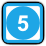Power Of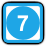Sin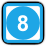Cosine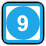Tangent